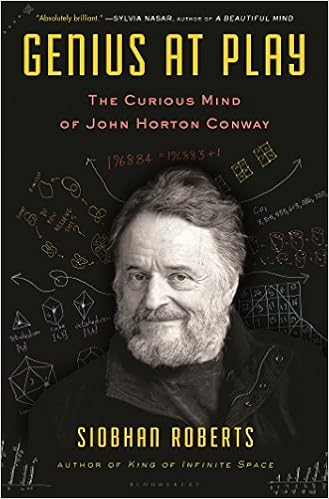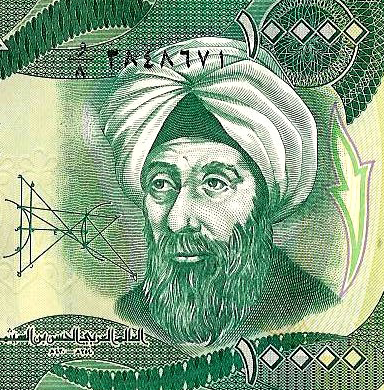# Mathspeak 101

### The language of mathematics

People use the word “math” in a variety of ways in popular culture. They say things like: “delegate math,” “the math doesn’t add up”, or my personal favorite: “do the math.”

But what do people really know about the subject? It is OK to be honest. There is no shame in not knowing much about math, despite what the Game of Thrones nun might say to the contrary.You non-mathematician readers are in for a treat. Welcome to your first taste of conversational mathematics! I call it Mathspeak 101.

### What is math, anyways?

Mathematics is about structure and the classification of structure. Structures are limitless, and broadly deal with things such as numbers, shapes (geometry), manipulating symbols and rules that govern their interaction (algebra and logic) or processes that change over time (analysis and dynamical systems).

Consider numbers for a minute.

Numbers are the most familiar structures in mathematics. We learn about them as children, and are taught how they combine through arithmetic operations such as addition and multiplication. There is, however, so much more to numbers than most of us consider. Numbers come in different forms, like integers, rationals, real numbers or complex numbers. Number theory studies these structures in a formal way, usually (but not always) with a view towards properties of the seemingly innocent-looking integers.

Within the integers live the primes: numbers that are only divisible by themselves or 1.The first 100 positive integers, with the primes highlighted.

Every number is a product of primes, and this product is unique up to the order of its factors. For example, 6 = 2 x 3 =3 x 2. Think of the primes as an infinite periodic table of elements, with the primes as building blocks of the integers.

We know there are infinitely many primes, but we don’t really understand them fully or how they are distributed.  For example, the Riemann Hypothesis is a statement about complex numbers with huge implications about the primes. The Riemann Hypothesis, by the way,  is one of deepest problems in all of mathematics. Or consider the abc conjecture which shows how little we understand about the interaction of the basic arithmetic operations.  Number theory has found applications in modern cryptography, which protects our banking systems.

Mathematics is not just about numbers, of course. Numbers are the tip of the iceberg of the structures we study, although they certainly are among the most beautiful and useful.

### What are proofs?

A proof, simply stated, is a sequence of logical propositions arguing the validity of some mathematical assertion. Confused? That’s OK. Proofs can confuse even the best mathematicians.

Bertrand Russell famously said that mathematics is about statements of the form: If P then Q. We are given some set of hypotheses P, and we claim that this implies the truth of the conclusion Q. Getting from P to Q is the tough part, and that is where proofs come in.

I like to tell my students that proofs are like an essay: they have an introduction, body, and conclusion. Or they can be viewed as a conversation between you and the ultimate sceptic. We claim a truth in theorem, and proofs explain why the truth holds. Every line of the proof must follow from previous assertions by clearly expounded logic and calculation, or the proof is incorrect or contains a gap.

Proofs come in all sizes. Some are brief, like the proof that the square root of 2 is irrational.  Then there is the proof of the classification of finite simple groups, which spans some 10,000 pages of highly non-trivial mathematics. There is no limit on the size of a proof, but mathematicians look for the most economical proofs.

Mathematicians are wary of ugly proofs, just like a classical violinist hates hearing industrial music. When the threads of a proof are simple, elegant, and connect seamlessly, that is when mathematicians are the most happy.

### Isn’t Mathematics finished?

No! Absolutely not.

Humans have studied mathematics for thousands of years. For every theorem we conquer, there are infinitely many unproven ones that beckon to us. One of the most interesting things about mathematics is that we don’t even know all the right questions. Every day new questions emerge, with only a fraction of them answered.

There are 97 subjects itemized by the Mathematics Subject Classification. From number theory to harmonic analysis to fluid dynamics, there are a huge number of diverse subjects studied in modern mathematics. At Euclid’s time or even Newton’s, one might claim to have mastered all known mathematics. Today, that would be utterly hopeless. Such giants like Paul Erdős or Emmy Noether had a deep understanding of several disparate fields of mathematics, but such intellects are far and few between.Emmy Noether (1882-1935). Generations of mathematicians were inspired by her work ranging from algebra to conservation laws in physics.

### What do mathematicians do?

We do proofs. We attempt to classify, explain, and unify structures and their properties. We also do numerical experiments, run algorithms, and use the tools of big data to mine properties of mathematical structures. We propose conjectures, scratch chalk on blackboards, and drink coffee while doing all of the above.

My own story: I do discrete mathematics or graph theory. I study networks, which capture relationships between objects. From social networks, to networks of proteins, to networks of web pages and their links, networks are everywhere in nature and technology. Graph theory is the abstract study of such networks.A visualisation of a social network.

### Why do people do mathematical research in the first place?

Mathematicians look for new patterns and try to explain existing ones. There is something majestic about it. As the famed mathematician John Conway said, you don’t need a spaceship or a giant particle accelerator to study mathematics. You just need to sit at your desk with paper and pen/pencil and think.

Sorry trees, but there is more work to do!John Conway, the subject of Siobhan Robert’s wonderful recent biography.

We are drawn to the universality of mathematics and to its undeniable beauty. So long as humans have the space and time to think about structures, mathematical thinking will organically emerge.

### Is mathematics really a language?

While mathematics is not a language like English or Mandarin, it has a syntax and an aesthetic. As humans, we are finite and imperfect. We make mistakes, and have a limited view of the universe of patterns around us.

Here is a quote from the polymath Alhazen (circa 965 – 1040):

“The seeker after the truth is not one who studies the writings of the ancients and, following his natural disposition, puts his trust in them, but rather the one who suspects his faith in them and questions what he gathers from them, the one who submits to argument and demonstration, and not to the sayings of a human being whose nature is fraught with all kinds of imperfection and deficiency. “Alhazen as pictured on an Iraqi bank-note.

Mathematics gives us a connection to the infinite and a momentary glimpse of perfection. It is a unique language that each new generation of explorers learns and expands upon.

Mathematics is a growing language, whose words and stories are infinite.

Next time you talk to a mathematician, don’t tell them you hate math or it was your worst subject in high school. Instead, tell them you passed Mathspeak 101 and say “Math is a universal language. Want to chat?”

Anthony Bonato